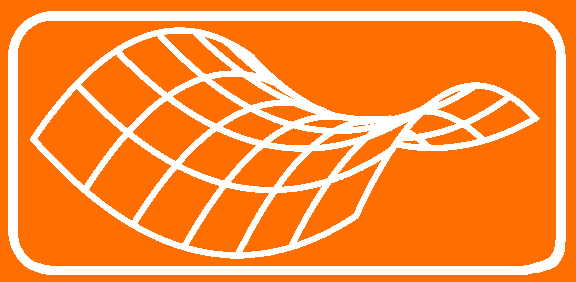Journal Home Page Cumulative Index List of all Volumes Complete Contentsof this Volume Previous Article Minimax Theory and its Applications 04 (2019), No. 1, 113--149Copyright Heldermann Verlag 2019 Existence of Periodic Orbits Near Heteroclinic Connections Giorgio Fusco Dip. di Matematica Pura ed Applicata, Universitŕ dell'Aquila, Via Vetoio, 67010 Coppito - L’Aquila, Italy fusco@univaq.it Giovanni F. Gronchi Dip. di Matematica, Universitŕ di Pisa, Largo B. Pontecorvo 5, 56127 Pisa, Italy giovanni.federico.gronchi@unipi.it Matteo Novaga Dip. di Matematica, Universitŕ di Pisa, Largo B. Pontecorvo 5, 56127 Pisa, Italy matteo.novaga@unipi.it [Abstract-pdf] \newcommand{\R}{\mathbb{R}} We consider a potential $W\colon \R^m\rightarrow\R$ with two different global minima $a_-, a_+$ and, under a symmetry assumption, we use a variational approach to show that the Hamiltonian system\\[2mm] \centerline{\hfill $\ddot{u}=W_u(u)$, \hfill(*)}\par has a family of $T$-periodic solutions $u^T$ which, along a sequence $T_j\rightarrow+\infty$, converges locally to a heteroclinic solution that connects $a_-$ to $a_+$. We then focus on the elliptic system\\[2mm] \centerline{\hfill $\Delta u=W_u(u),\;\; u\colon \R^2\rightarrow\R^m$, \hfill(**)}\par that we interpret as an infinite dimensional analogous of (*), where $x$ plays the role of time and $W$ is replaced by the action functional $J_\R(u)=\int_\R(\frac{1}{2}\vert u_y\vert^2+W(u))dy$. We assume that $J_\R$ has two different global minimizers $\bar{u}_-, \bar{u}_+\colon \R\rightarrow\R^m$ in the set of maps that connect $a_-$ to $a_+$. We work in a symmetric context and prove, via a minimization procedure, that (**) has a family of solutions $u^L\colon \R^2\rightarrow\R^m$, which is $L$-periodic in $x$, converges to $a_\pm$ as $y\rightarrow\pm\infty$ and, along a sequence $L_j\rightarrow+\infty$, converges locally to a heteroclinic solution that connects $\bar{u}_-$ to $\bar{u}_+$. Keywords: Action-minimizing solutions, periodic orbits, homoclinic orbits, heteroclinic orbits, variational methods. MSC: 37J50, 37J45 [ Fulltext-pdf  (240  KB)] for subscribers only.